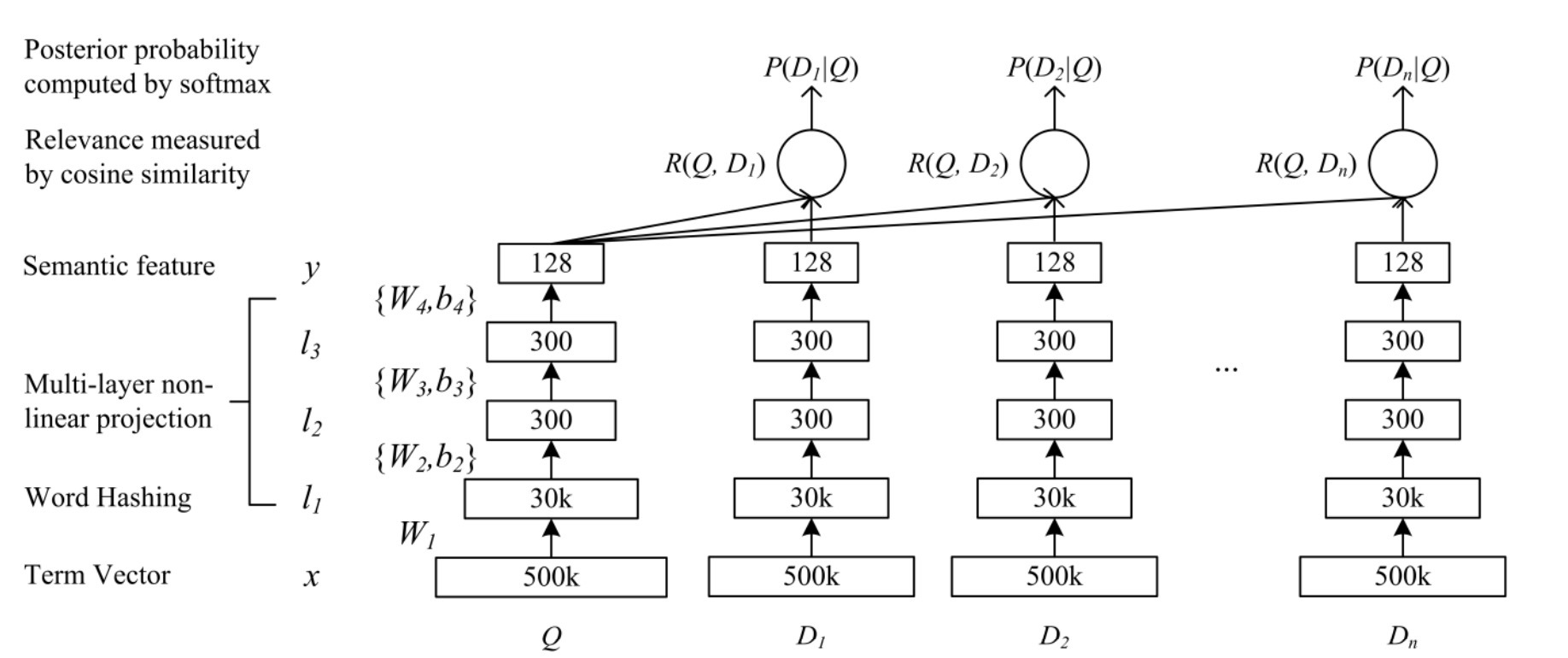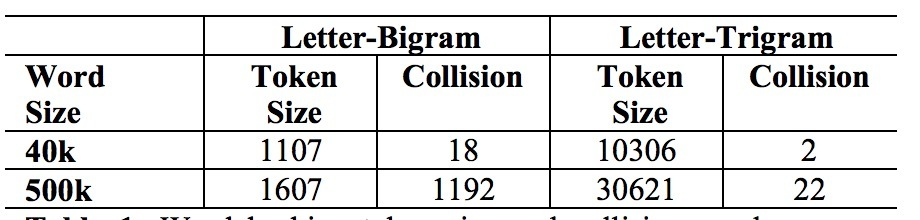DSSM介绍

April 04, 2017

microsoft在2013年提出了DSSM结构：《Learning Deep Structured Semantic Models for Web Search using Clickthrough Data》。我们来看下该paper。

介绍

2.1 隐语义模型和点击数据的使用

IR领域最著名的线性投影模型是LSA。通过在一个document-term矩阵上使用SVD，一个document(或一个query)可以映射成一个低维概念向量(conecpt vector)：$\hat{D} = A^T D$，其中A是投影矩阵。在文档搜索中，在一个query和一个document间的相关分(可以通过term向量分别表示为Q和D)，根据投影矩阵A， 被认为是与概念向量$\hat{Q}$和$\hat{D}$间的cosine相似度得分成比例：

$sim_A(Q,D) = \frac{\hat{Q}^T \hat{D}}{ \| \hat{Q} \| \|\hat{D} \|}$

…(1)

3.DSSM

3.1 DNN用于计算语义特征• 1) 将term vectors映射到它们相应的语义concept vectors上
• 2) 计算一个document和一个query间相关分，作为他们相应的语义concept vectors的cosine相似度 等式(3)和(5)

$l_1 = W_1 x \\ l_i = f(W_i l_{i-1} + b_i), i=2, ..., N-1 \\ y = f(W_N l_{N-1} + b_N)$

…(3)

$f(x)= \frac{1 - e^{-2x}}{1 + e^{-2x}}$

…(4)

$R(Q,D) = cosine(y_Q, y_D) = \frac{y_Q^T y_D}{ \| y_Q \| \| y_D \|}$

…(5)

3.2 word hashing3.3 DSSM的学习

$P(D|Q) = \frac{exp(\gamma R(Q,D))}{\sum_{D' \in D} exp(\gamma R(Q,D'))}$

…(6)

• Q是一个query
• $D^+$是点击的文档
• ${D_j^-; j=1, ..., 4}$表示4个随机选中的未点击文档。

$L(\Lambda) = -log \prod\limits_{Q,D^+} P(D^+ | Q)$

…(7)

参考

Updated on

STAR算法介绍

PLE介绍

Published on March 04, 2021

DTS介绍

Published on January 02, 2021